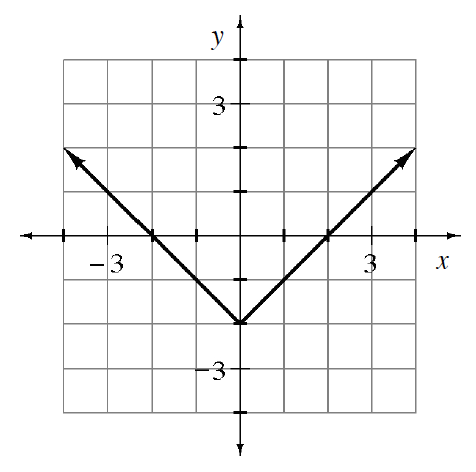Home > APCALC > Chapter 1 > Lesson 1.4.1 > Problem1-140

1-140.

The graph of $y = f(x)$ is sketched at right.

Start by making a table of values for the given function.

1. Sketch $y = f(−x)$.

Give each of the $x$-values in your table the opposite sign and graph the new function.

2. Sketch $y = −f(x)$.

Since $f(x) = y$, give each of the $y$-values in your original table the opposite sign. Then graph the new function.

3. Sketch $y = f(f(x))$.

Add a third column to your original table labeled $f(f(x))$. Then, for example, $f(f(–3)) = f(1) = -1$.